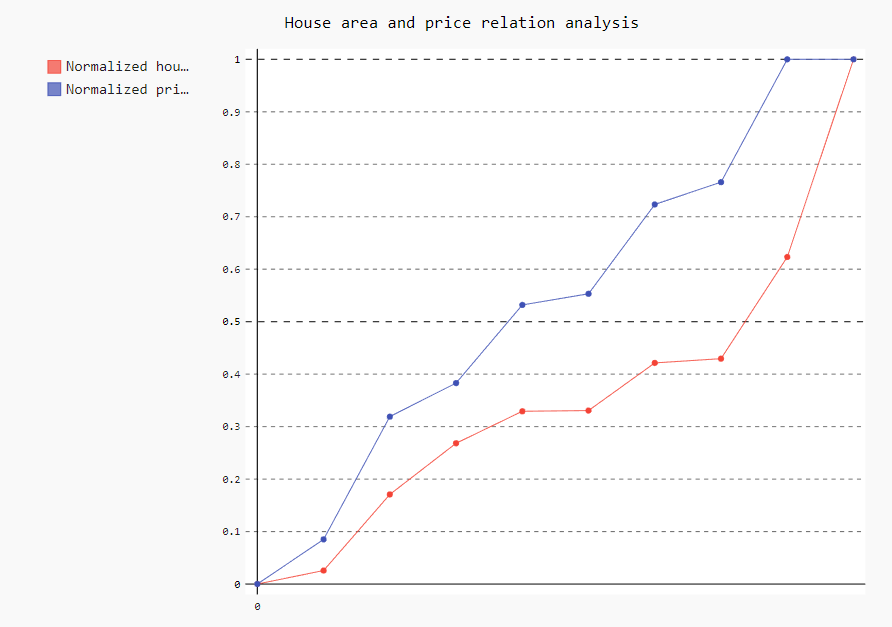# Pygal Tutorial: Part 1# Pygal Tutorial: Part 1

Hi ML Enthusiasts! Today, in this part 1 of Pygal tutorial, we will be working on visualizing data using Pygal plots. From today, I will be introducing new feature to my blog posts, i.e., explaining Python codes and concepts through Jupyter notebooks. They are great for step-by-step analysis of the codes as well as the concepts. So, this is how it goes:

### Visualizing data using Pygal

Pygal is a data visualization library developed by Kozea community. One great thing about Pygal is that it creates graphs and bar charts in SVG or Scalable Vector Graphics format. This makes the charts great for any kind of print media as there is no issue of losing image quality due to pixel related issues or so.

### Pygal installation

Installing Pygal requires lxml library as a pre-requisite and then Pygal can be installed using pip. For doing so, run the following commands:

conda install lxml

pip install pygal

### Importing Pygal

Next thing to be done is to import the pygal library in our notebook and setting an alias ‘py’ for it. This can be done by the following code:

In :
```import pygal as py
```

### Line charts in Pygal

Now, let’s learn how to create a line chart using pygal. For doing so, we use the Line() function of Pygal. We use this by creating an object of pygal.Line() and using that object, we create the title, x-labels, y-labels etc for that chart. The code for creating the line chart is given below:

In :
```line = py.Line()
line.title = "Salary variation of Shweta in the past 5 years"    #Set the title of the line chart
line.x_labels = (2014, 2015, 2016, 2017, 2018)   #Setting the labels for x-axis
line.add('Salary in lakhs in INR', [None, 3, 5, 8, 10, 15])    #Setting the salary values to be plotted on graph
line.render_to_file("salary_variation.svg")    #This saves the chart in the current library in the file salary_year_variation.svg
stacked_line.render_in_browser()
```
```file://C:/Users/jyoti/AppData/Local/Temp/tmppvm9w7bt.html
```

The chart will get created and will be stored in a file named as “salary_year_variation.svg” in the library your jupyter notebook is saved. You can go and open the file with Google Chrome. Notice that when you hover over the line, the line becomes bold. When you hover over the points, you will be able to see the description of the points getting popped up.

### Stacked line charts using Pygal

Suppose we are given multiple data series and we want to plot the line charts for all of them. We can do this in two ways. First way will be to make line chart for each data series but there is a possibility that we can have difficulty if we want to compare contribution from each of them. So, we can think of second way too. Second way will be to add multiple line charts on a single chart, that is, include the contribution of all of them in a single chart and call it as stacked line chart.

The code for the same is given below:

In :
```import numpy as np    #importing numpy package with alias as 'np'
import pandas as pd    #importing pandas package with alias as 'pd'
```

We will do the analysis on house area vs price of houses based on their areas. We will be preparing our own datasets in this case. First, by using the random module of numpy, we will generate house_area in square feet with lowest value being 1000 sq. ft. and highest area being 1800 sq.ft.(Suppose a locality has house areas in this range only). This generates an array of arrays. To make the manipulations easier, we will use list() function to convert the array of arrays into list of arrays. The code for this is given below:

### Generating house_area array

In :
```house_area = list(np.random.randint(low= 1000, high= 1800, size= (10,1)))
house_area
```
Out:
```[array(),
array(),
array(),
array(),
array(),
array(),
array(),
array(),
array(),
array()]```

Now, we will convert this list of arrays into list of floats using float() function. Please note that we have used list comprehension in both the steps. The output we get for the same is given below:

In :
```house_area = [float(l) for l in house_area]
house_area
```
Out:
```[1186.0,
1798.0,
1377.0,
1258.0,
1371.0,
1079.0,
1520.0,
1303.0,
1304.0,
1060.0]```

### Sorted function

Now, we will sort the house_area by using sorted() function.

In :
```house_area = sorted(house_area)
house_area
```
Out:
```[1060.0,
1079.0,
1186.0,
1258.0,
1303.0,
1304.0,
1371.0,
1377.0,
1520.0,
1798.0]```

### Generating price in lakhs

Now that we have generated house_area, we will generate the random prices for them.

In :
```price_in_lakhs = list(np.random.randint(low= 50, high= 100, size= (10,1)))
price_in_lakhs
```
Out:
```[array(),
array(),
array(),
array(),
array(),
array(),
array(),
array(),
array(),
array()]```
In :
```price_in_lakhs = [float(l) for l in price_in_lakhs]
price_in_lakhs
```
Out:
`[70.0, 77.0, 99.0, 56.0, 52.0, 88.0, 67.0, 99.0, 86.0, 78.0]`
In :
```price_in_lakhs = sorted(price_in_lakhs)
price_in_lakhs
```
Out:
`[52.0, 56.0, 67.0, 70.0, 77.0, 78.0, 86.0, 88.0, 99.0, 99.0]`

### Normalizing the data

Now that we have both of them ready, let’s normalize them in order to make the comparison easy. The formula for normalisation is
x_normalised = (x-min(x))/range(x).
Since the list is sorted, Max(x) can be found by using x-1 and min(x) can be found using x0. Range(x) = max(x) – min(x). The code for this is as follows:

In :
```range_house_area = house_area[-1] - house_area
normalized_house_area = [(l - house_area)/range_house_area for l in house_area]
normalized_house_area
```
Out:
```[0.0,
0.025745257452574527,
0.17073170731707318,
0.2682926829268293,
0.32926829268292684,
0.33062330623306235,
0.42140921409214094,
0.42953929539295393,
0.6233062330623306,
1.0]```
In :
```range_price_in_lakhs = price_in_lakhs[-1] - price_in_lakhs
normalized_price_in_lakhs = [(l - price_in_lakhs)/range_price_in_lakhs for l in price_in_lakhs]
normalized_price_in_lakhs
```
Out:
```[0.0,
0.0851063829787234,
0.3191489361702128,
0.3829787234042553,
0.5319148936170213,
0.5531914893617021,
0.723404255319149,
0.7659574468085106,
1.0,
1.0]```

### Obtaining the plot

Now that we have both the variables/features ready, let’s make stacked line chart for both of them. The code for this is as follows:

In :
```stacked_line = py.Line()
stacked_line.title = "House area and price relation analysis"
stacked_line.x_labels = map(str, range(0, 1))    #Setting the x-axis range from 0 to 1 and converting them into string.
```file://C:/Users/jyoti/AppData/Local/Temp/tmpizyqrmkf.html